Next: Mathematical Background Up: The Schrödinger Equation Previous: The Time-Independent Schrödinger Equation

## The Time-Dependent Schrödinger Equation

We are now ready to consider the time-dependent Schrödinger equation. Although we were able to derive the single-particle time-independent Schrödinger equation starting from the classical wave equation and the de Broglie relation, the time-dependent Schrödinger equation cannot be derived using elementary methods and is generally given as a postulate of quantum mechanics. It is possible to show that the time-dependent equation is at least reasonable if not derivable, but the arguments are rather involved (cf. Merzbacher , Section 3.2; Levine , Section 1.4).

The single-particle three-dimensional time-dependent Schrödinger equation is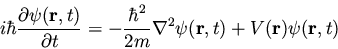(21)

where V is assumed to be a real function and represents the potential energy of the system (a complex function V will act as a source or sink for probability, as shown in Merzbacher , problem 4.1). Wave Mechanics is the branch of quantum mechanics with equation (21) as its dynamical law. Note that equation (21) does not yet account for spin or relativistic effects.

Of course the time-dependent equation can be used to derive the time-independent equation. If we write the wavefunction as a product of spatial and temporal terms,, then equation (21) becomes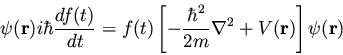(22)

or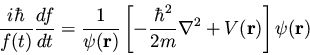(23)

Since the left-hand side is a function of t only and the right hand side is a function of
only, the two sides must equal a constant. If we tentatively designate this constant E (since the right-hand side clearly must have the dimensions of energy), then we extract two ordinary differential equations, namely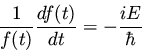(24)

and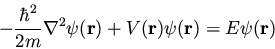(25)

The latter equation is once again the time-independent Schrödinger equation. The former equation is easily solved to yield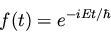(26)

The Hamiltonian in equation (25) is a Hermitian operator, and the eigenvalues of a Hermitian operator must be real, so E is real. This means that the solutions f(t) are purely oscillatory, since f(t) never changes in magnitude (recall Euler's formula
). Thus if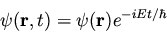(27)

then the total wave function
differs from
only by a phase factor of constant magnitude. There are some interesting consequences of this. First of all, the quantity
is time independent, as we can easily show: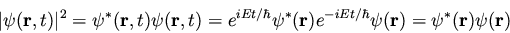(28)

Secondly, the expectation value for any time-independent operator is also time-independent, ifsatisfies equation (27). By the same reasoning applied above,
(29)

For these reasons, wave functions of the form (27) are called stationary states. The stateis stationary,'' but the particle it describes is not!

Of course equation (27) represents a particular solution to equation (21). The general solution to equation (21) will be a linear combination of these particular solutions, i.e.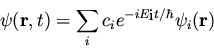(30)Next: Mathematical Background Up: The Schrödinger Equation Previous: The Time-Independent Schrödinger Equation